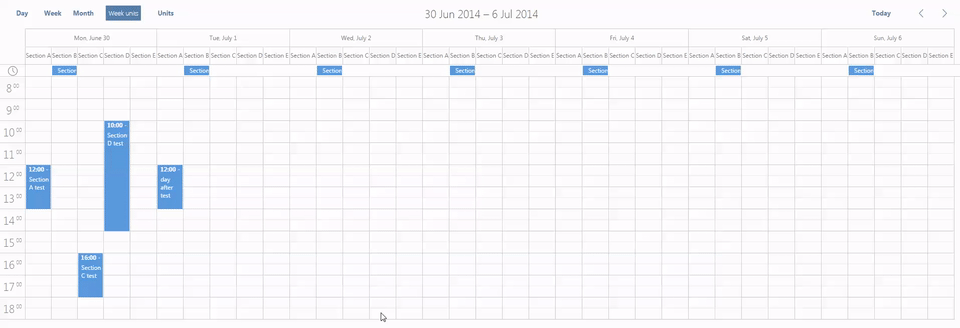# wrong event section in multiday unit view

Hello ,

we are currently using scheduler v4.3.17 commercial licence.

and our clients started complaining about an issue where the events are created in the wrong section.
for example i click section “b” it get created in “c” or “d”.
i found out that the event during it’s creation doesnt have the section id so to fix it i added this line to the function scheduler.addEventNow

```base.id = this._drag_id = this.uid(); var pr = this._props[this._mode]; if(pr && e){ base[this._props[this._mode].map_to] = this.getActionData(e).section ; } ```
now the event is created where it should . but there’s a new issue , if we create a view unit that has 7 days 7 units , the event is created in wrong section , i think there’s is a problem with the way how it’s calculated ,
i tracked the issue to the function scheduler[“mouse_”+ name] = function(pos){ //mouse_coord handler in dhtmlxscheduler_units.js.

this issue is also in v4.3.35.

[code]<!doctype html>

Multiday Units view html, body{ margin:0px; padding:0px; height:100%; overflow:hidden; }

[/code]

Thank you.

fixed the issue , some columns were missing 1px (37px others 38px ) the issue was in this line ``` column = x_pos / width; ```

[code]scheduler._get_column_index = function(x_pos) {
var column = 0;
if (this._cols) {

``````    var width = 0;
for (var i = 0; i < this._cols.length && !width; i++) {
width = this._cols[i];
}

if (width) {

//column = x_pos / width;
// youssef : not accurate way to calculate sections , sometimes 1px is missing
var x = 0;
for(var j=0; this._cols.length ;j++){
if( x <= x_pos ){
x+= this._cols[j];
}else{
column = j;
break;
}
}

} else {
column = 0;
}

if (this._ignores)
for (var i = 0; i <= column; i++)
if (this._ignores[i])
column++;
}
return column;
``````

};[/code]

what do you think of this solution ?

here’s the issue , to recreate the bug all you have to do is create 7 days and 5 or 7 units .